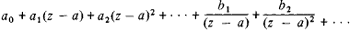# Laurent Series

Also found in: Wikipedia.

## Laurent series

[lȯ′ränz ‚sir·ēz]
(mathematics)

## Laurent Series

a series of the form(*)

that is, a series of positive and negative powers of the difference z — a (where z, a, and the coefficients of the series are complex numbers). Here, the nonnegative powers form an ordinary power series that converges, in general, within a circle with center a and radius R ≤ ∞. The remaining terms form a series that converges, in general, outside a circle with the same center but with radius r ≥ 0. If r < R, then the series (*) converges in the annulus r < ǀz — a < R to an analytic function of the complex variable z.

Although series of the form (*) were already known to L. Euler (1748), they were named after P. Laurent, who in 1843 proved that every function of a complex variable, single-valued and analytic in the annulus r < |z — | < R, can be expanded in this annulus in this type of series (Laurent theorem). The theorem, however, was obtained somewhat earlier by K. Weierstrass in a work that remained unpublished until 1894.

References in periodicals archive ?
Among their topics are holomorphic functions, Taylor and Laurent series, spaces of functions, Fourier analysis, and linear operators in Hilbert spaces: the finite-dimension and infinite-dimensional cases.
Because the conformal transformation function z([zeta]) is analytic in the ring D" bounded by the circles [absolute value of [zeta] = 1] and [absolute value of [zeta] = r], the functions [phi](z) and [psi](0, which must be analytic throughout D", can be expanded in the form of Laurent series 
This means that they can be represented by their Laurent series expansions .
It is known, see, for example, , that E (s; k/l, 0) has the Laurent series expansion
All of the computed examples in this paper, except for the Laurent series example in the last section, are performed using variations of this reflection algorithm.
Chapters cover the complex plane, complex line integrals, applications of the Cauchy theory, Laurent series, the argument principle, the geometric theory, harmonic functions, infinite series and products, and analytic continuation.
Laurent series, the ships remained in service in their original condition, although they did receive an austere refit under the Destroyer Life Extension Program (DELEX), in the mid-eighties.
The methodology [Gil, 1995] that underlies this proposal is based on a generalization of the PA for formal Laurent series [Bultheel, 1987].
They cover complex numbers and their geometry, complex differentiability, Cauchy integral theorem and its consequences, Taylor and Laurent series, and harmonic functions.
They begin by describing complex numbers and their elementary properties, including power series, powers and logarithms and the geometric properties of simple functions, analytic functions such as differentiation and integration in the complex frame, Cauchy's integral formula, Taylor and Laurent series and analytic continuation, contour integration, conformal mapping, including the Joukowsky and Schwarz-Christoffel transformations, special functions such as the gamma function and the Lefendre and Bessel functions, asymptotic methods such as that of Laplace, transform methods such as Fourier transforms, and special techniques such as the Weiner-Hopf methods, the kernel decomposition and using approximate kernels.
It also discusses elementary aspects of complex analysis such as the Cauchy integral theorem, the residue theorem, Laurent series, the Riemann mapping theorem, and more advanced material selected from Riemann surface theory.

Site: Follow: Share:
Open / Close